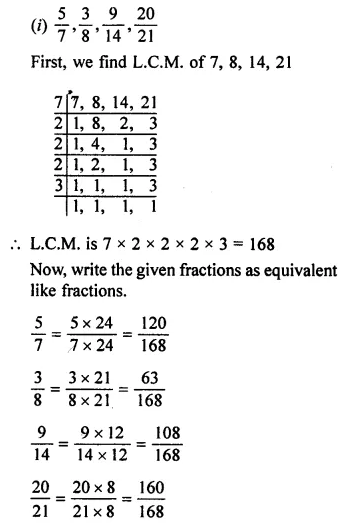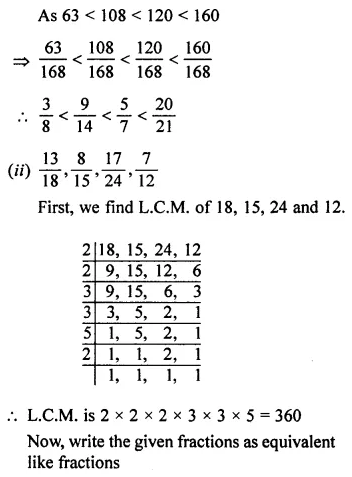## ML Aggarwal Class 7 Solutions for ICSE Maths Chapter 2 Fractions and Decimals Ex 2.1

Question 1.
What fraction of each of the following figure is shaded?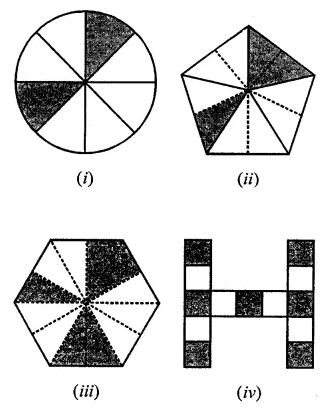Solution:
(i) Fraction is $$\frac { 2 }{ 8 }$$ = $$\frac { 1 }{ 4 }$$
(ii) Fraction is $$\frac { 3 }{ 10 }$$
(iii) Fraction is $$\frac { 5 }{ 12 }$$
(iv) Fraction is $$\frac { 7 }{ 13 }$$

Question 2.
What fraction of an hour is 35 minutes?
Solution:
(i) 1 hour = 60 minutes
Fraction = $$\frac { 35 }{ 60 }$$ = $$\frac { 7 }{ 12 }$$

Question 3.
Convert the following fractions into improper fractions :
(i) 2$$\frac { 7 }{ 9 }$$
(ii) 5$$\frac { 4 }{ 11 }$$
Solution:Question 4.
Convert the following fractions into mixed fractions:
(i) $$\frac { 73 }{ 8 }$$
(ii) $$\frac { 94 }{ 13 }$$
Solution:Question 5.
Fill in the missing numbers in the following equivalent fractions:
(i) $$\frac { 3 }{ 7 }$$ = $$\frac { …. }{ 35 }$$
(ii) $$\frac { 5 }{ …. }$$ = $$\frac { 30 }{ 18 }$$
(iii) $$\frac { ….. }{ 9 }$$ = $$\frac { 56 }{ 72 }$$
Solution
(i) $$\frac { 3 }{ 7 }$$ = $$\frac { …. }{ 35 }$$
The denominator in the second fraction is 35.
To get 35 from 7, we have to multiply 7 by 5.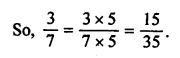(ii) $$\frac { 5 }{ …. }$$ = $$\frac { 30 }{ 18 }$$
To make both fractions equal, we multiply the numerator of the first fraction by 5.
The numerator itx the first fraction is 5. To get 5 from 30, we have to divide 30 ÷ 6.
To make both fractions equal, we divide denominator of the second fraction by 6.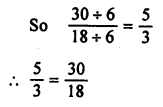(iii) $$\frac { ….. }{ 9 }$$ = $$\frac { 56 }{ 72 }$$
The denominator in the second fraction is 72 and the denominator in the first fraction is 9.
To get 9 from 72, we have to divide 72 ÷ 8
To make both fractions equal, we divide the numerator of the second fraction by 8.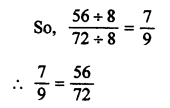Question 6.
Reduce the following fractions to their simplest form:
(i) $$\frac { 48 }{ 72 }$$
(ii) $$\frac { 276 }{ 115 }$$
(iii) $$\frac { 72 }{ 336 }$$
Solution:Question 7.
Convert the following fractions into equivalent like fractions:Solution: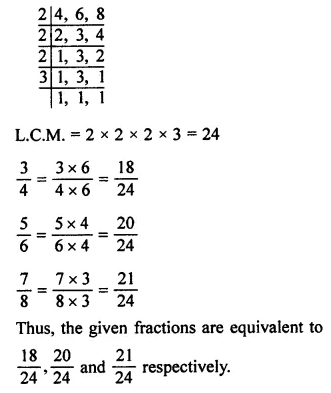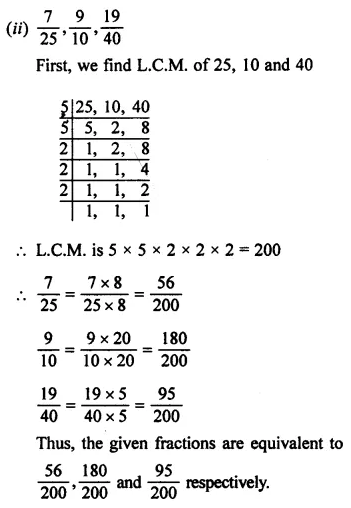Question 8.
Arrange the given fractions in descending order: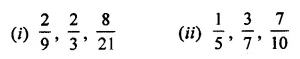Solution: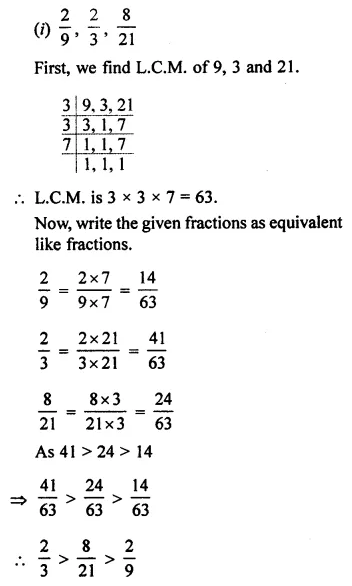Question 9.
Arrange the given fractions in ascending order:Solution: